# Algebra 3/4 : Basic Trigonometry

## Example Questions

### Example Question #1 : Basic Trigonometry

What is the degree equivalent for an angle that is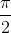radians?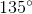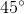Explanation:

To find the degree equivalent for an angle that isradians first recall the unit conversion between degrees and radians.

There are 360 degrees orradians in a circle.

When simplified this is,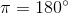therefore to convert from radians to degrees, simply multiply by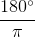.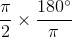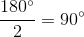### Example Question #2 : Basic Trigonometry

What is the degree equivalent for an angle that is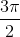radians?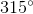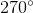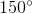Explanation:

To find the degree equivalent for an angle that isradians first recall the unit conversion between degrees and radians.

There are 360 degrees orradians in a circle.

When simplified this is,therefore to convert from radians to degrees, simply multiply by.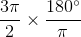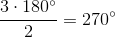### Example Question #21 : Algebra 3/4

What is the degree equivalent for an angle that isradians?Explanation:

To find the degree equivalent for an angle that isradians first recall the unit conversion between degrees and radians.

There are 360 degrees orradians in a circle.

When simplified this is,therefore to convert from radians to degrees, simply multiply by.### Example Question #4 : Basic Trigonometry

What is the degree equivalent for an angle that isradians?Explanation:

To find the degree equivalent for an angle that isradians first recall the unit conversion between degrees and radians.

There are 360 degrees orradians in a circle.

When simplified this is,therefore to convert from radians to degrees, simply multiply by.### All Algebra 3/4 Resources